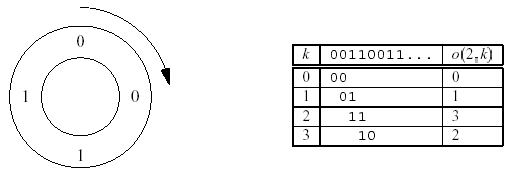Online JudgeProblem SetAuthorsOnline ContestsUser
Web Board
F.A.Qs
Statistical Charts
Problems
Submit Problem
Online Status
Prob.ID:
Register
Authors ranklist
Current Contest
Past Contests
Scheduled Contests
Award Contest
Register
Language:
Ouroboros Snake
 Time Limit: 1000MS Memory Limit: 65536K Total Submissions: 1532 Accepted: 778

Description

Ouroboros is a mythical snake from ancient Egypt. It has its tail in its mouth and continously devours itself.

The Ouroboros numbers are binary numbers of 2^n bits that have the property of "generating" the whole set of numbers from 0 to 2^n - 1. The generation works as follows: given an Ouroboros number, we place its 2^n bits wrapped in a circle. Then, we can take 2^n groups of n bits starting each time with the next bit in the circle. Such circles are called Ouroboros circles for the number n. We will work only with the smallest
Ouroboros number for each n.

Example: for n = 2, there are only four Ouroboros numbers. These are 0011;0110;1100; and 1001. In this case, the smallest one is 0011. Here is the Ouroboros circle for 0011:The table describes the function o(n;k) which calculates the k-th number in the Ouroboros circle of the smallest Ouroboros number of size n. This function is what your program should compute.

Input

The input consists of several test cases. For each test case, there will be a line containing two integers n and k (1<=n<=15; 0<=k<2^n). The end of the input file is indicated by a line containing two zeros. Don抰 process that line.

Output

For each test case, output o(n;k) on a line by itself.

Sample Input

```2 0
2 1
2 2
2 3
0 0```

Sample Output

```0
1
3
2```

Source

[Submit]   [Go Back]   [Status]   [Discuss]Home PageGo BackTo top